# Using Gauss law find out electric field intensity due to a line charge

Using Gauss law find out electric field intensity due to a line charge.

A line charge is in the form of a thin charged rod with linear charge density λ. Let us find out an expression for electric intensity at any point P at a perpendicular distance r from the rod.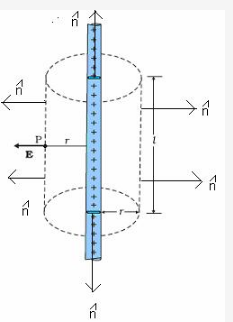Let us consider a right circular closed cylinder of radius r and length l, with infinitely long line of charge as its axis.
The magnitude of electric field intensity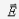at every point on the curved surface of the cylinder is same, because all such points are at the same distance from the line charge.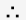Contribution of curved surface of cylinder towards electric flux,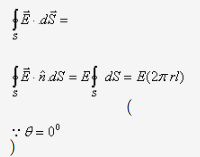Whereis the curved surface area of cylinder.
On the ends of the cylinder angle betweenand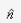is 90
. Therefore these ends make no contribution to the electric flux of the cylinder.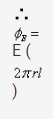Charge enclosed in the cylinder= linear charge density x length = λl
According to Gauss’s theorem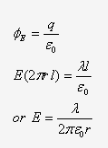The direction of electric field vector will be the function of whether the line charge is positive or negative
If λ < 0, i.e., in a negatively charged wire, the direction of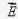is radially inward towards the wire.
If λ > 0, i.e., in a positively charged wire, the direction ofis radially out of the wire.